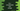# 4 ways in C++ to concatenate two strings## C++ program to concatenate two strings:

In this post, we will learn how to concatenate two strings in C++. We will write a program that will concatenate two strings and create a new string.

We can concatenate two strings in different ways in C++. I will show you different ways to do that in this post.

### Method 1: Using a for loop:

Let’s try this with a for loop. We can iterate through the characters of each string one by one and append them to a final result string.

This program will take the strings as inputs from the user:

``````#include <iostream>
using namespace std;

int main()
{
string firstStr, secondStr, resultStr;

cout << "Enter the first string: " << endl;
cin >> firstStr;

cout << "Enter the second string: " << endl;
cin >> secondStr;

for (int i = 0; i < firstStr.size(); i++)
{
resultStr += firstStr[i];
}

for (int i = 0; i < secondStr.size(); i++)
{
resultStr += secondStr[i];
}

cout << "Final string: " << resultStr << endl;

return 0;
}``````

Here,

• firstStr and secondStr variables are used to store the user input strings. resultStr is used to keep the final concatenate string.
• It reads the strings as inputs from the user.
• The two for loops are used to iterate through the characters one by one. It adds the characters to resultStr to make the final result string.

If you run this program, it will print output as like below:

``````Enter the first string:
hello
Enter the second string:
world
Final string: helloworld``````

We can also use a separate method to do the concatenation.

``````#include <iostream>
using namespace std;

string concatenateString(string firstStr, string secondStr)
{
string resultStr;

for (int i = 0; i < firstStr.size(); i++)
{
resultStr += firstStr[i];
}

for (int i = 0; i < secondStr.size(); i++)
{
resultStr += secondStr[i];
}

return resultStr;
}

int main()
{
string firstStr, secondStr;

cout << "Enter the first string: " << endl;
cin >> firstStr;

cout << "Enter the second string: " << endl;
cin >> secondStr;

string resultStr = concatenateString(firstStr, secondStr);

cout << "Final string: " << resultStr << endl;

return 0;
}``````
• concatenateString is the method that takes two strings and returns the concatenated string.

The advantage of using a separate method is that we can call this method from any other place. We have to write the method once and we can use it from multiple places.

### Method 2: By using a while loop:

We can also use a while loop to concatenate the strings. This will be similar to the above program.

``````#include <iostream>
using namespace std;

string concatenateString(string firstStr, string secondStr)
{
string resultStr;
int i = 0;

while (i < firstStr.size())
{
resultStr += firstStr[i];
i++;
}

i = 0;
while (i < secondStr.size())
{
resultStr += secondStr[i];
i++;
}

return resultStr;
}

int main()
{
string firstStr, secondStr;

cout << "Enter the first string: " << endl;
cin >> firstStr;

cout << "Enter the second string: " << endl;
cin >> secondStr;

string resultStr = concatenateString(firstStr, secondStr);

cout << "Final string: " << resultStr << endl;

return 0;
}``````
• Here, instead of for loops, we are using while loops in the concatenateString method.
• i is used for the while loops. So, before the second loop starts, we have to reset it to 0.

### Method 3: By using append():

The append method is an inbuilt method to append one string to the end of another string. It appends a string to the end of another string and returns that new string.

This method is defined as like below:

``string_1.append(string_2)``

Where string_1 and string_2 are the two strings. string_2 will be appended to the end of string_1. It returns the new string.

Let’s check it with an example:

``````#include <iostream>
using namespace std;

string concatenateString(string firstStr, string secondStr)
{
return firstStr.append(secondStr);
}

int main()
{
string firstStr, secondStr;

cout << "Enter the first string: " << endl;
cin >> firstStr;

cout << "Enter the second string: " << endl;
cin >> secondStr;

string resultStr = concatenateString(firstStr, secondStr);

cout << "Final string: " << resultStr << endl;

return 0;
}``````

I am changing the concatenateString method of the above example. This method returns the return value of the append method. It appends the second string to the end of the first string.

If you run this program, it will print output as like below:

``````Enter the first string:
hello
Enter the second string:
world
Final string: helloworld``````

### Method 4: Concatenate two C-strings by using the strcat method:

The strcat method is used to concatenate two c-strings or char* variables. you can use this method if you are not using string to hold the strings.

This method returns the concatenated string. It is defined as like below:

``strcat(char* str1, char* str2)``

Here, str1 and str2 are the two strings. This method appends str2 to the end of str1in placee.

Let’s try this method with an example:

``````#include <iostream>
using namespace std;

int main()
{
char firstStr[] = "hello";
char secondStr[] = "world";

strcat(firstStr, secondStr);

cout << "Final string: " << firstStr << endl;

return 0;
}``````

It will print the below output:

``Final string: helloworld``